# sklearn.datasets.make_circles¶

sklearn.datasets.make_circles(n_samples=100, *, shuffle=True, noise=None, random_state=None, factor=0.8)[source]

Make a large circle containing a smaller circle in 2d.

A simple toy dataset to visualize clustering and classification algorithms.

Read more in the User Guide.

Parameters:
n_samplesint or tuple of shape (2,), dtype=int, default=100

If int, it is the total number of points generated. For odd numbers, the inner circle will have one point more than the outer circle. If two-element tuple, number of points in outer circle and inner circle.

Changed in version 0.23: Added two-element tuple.

shufflebool, default=True

Whether to shuffle the samples.

noisefloat, default=None

Standard deviation of Gaussian noise added to the data.

random_stateint, RandomState instance or None, default=None

Determines random number generation for dataset shuffling and noise. Pass an int for reproducible output across multiple function calls. See Glossary.

factorfloat, default=.8

Scale factor between inner and outer circle in the range [0, 1).

Returns:
Xndarray of shape (n_samples, 2)

The generated samples.

yndarray of shape (n_samples,)

The integer labels (0 or 1) for class membership of each sample.

## Examples using sklearn.datasets.make_circles¶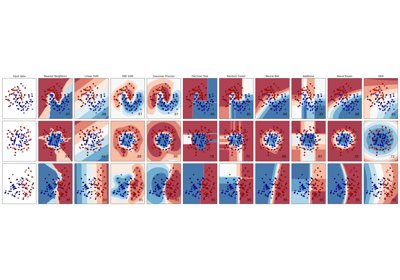Classifier comparison

Classifier comparison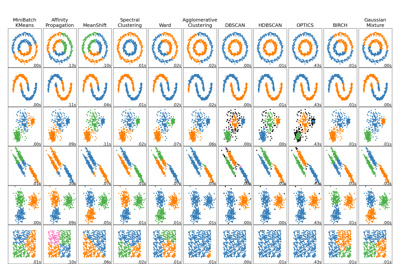Comparing different clustering algorithms on toy datasets

Comparing different clustering algorithms on toy datasets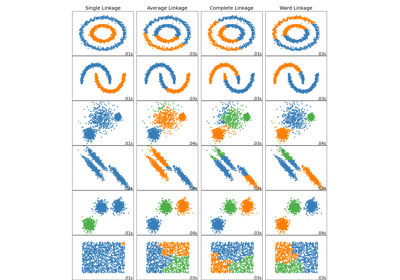Comparing different hierarchical linkage methods on toy datasets

Comparing different hierarchical linkage methods on toy datasets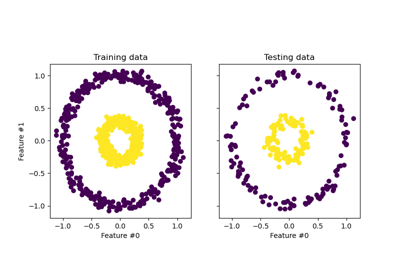Kernel PCA

Kernel PCA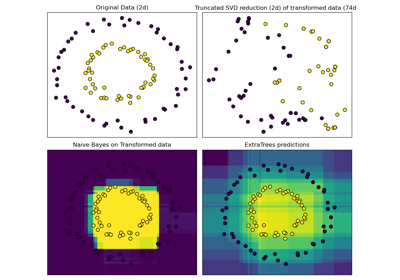Hashing feature transformation using Totally Random Trees

Hashing feature transformation using Totally Random Trees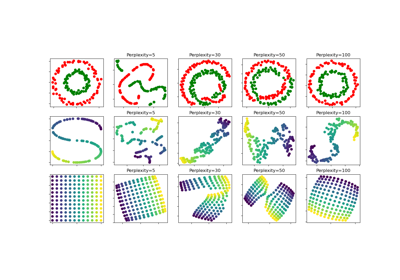t-SNE: The effect of various perplexity values on the shape

t-SNE: The effect of various perplexity values on the shape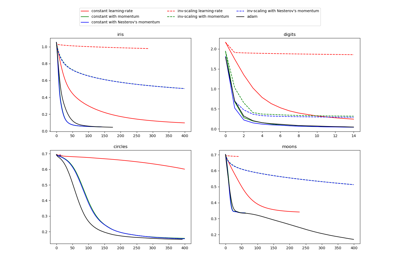Compare Stochastic learning strategies for MLPClassifier

Compare Stochastic learning strategies for MLPClassifier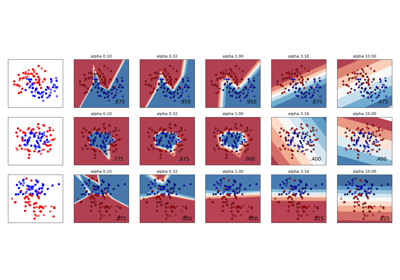Varying regularization in Multi-layer Perceptron

Varying regularization in Multi-layer Perceptron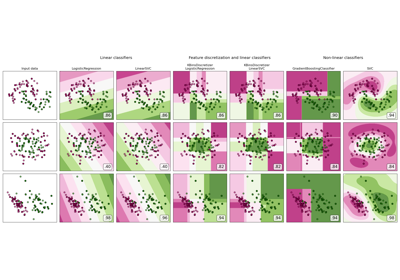Feature discretization

Feature discretization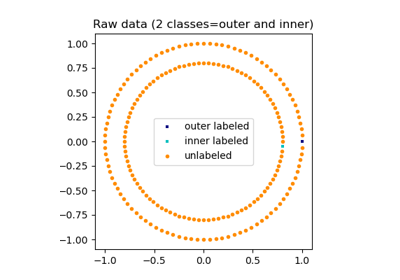Label Propagation learning a complex structure

Label Propagation learning a complex structure